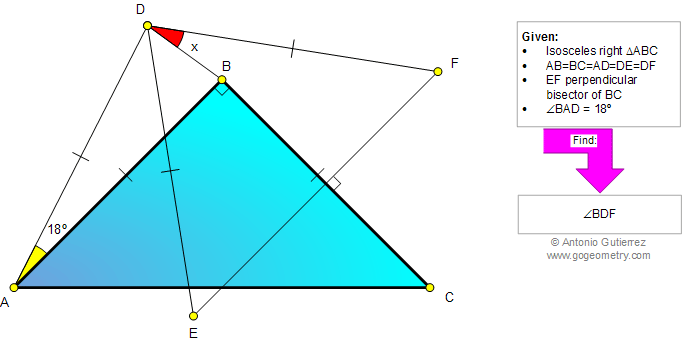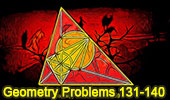# Problem 859: Isosceles Right Triangle, Angles,18, 45 Degrees, Congruence, Perpendicular bisector. Level: High School, SAT Prep, College geometry

 The figure shows an isosceles right triangle ABC. EF is the perpendicular bisector of BC, AB=BC=AD=DE=DF and angle BAD = 18 degrees. Find the measure of angle BDF. Problem submitted by Charles T.See More Geometry Problems:Geometry Problem 860 Triangle, Three Medians, Parallel, Parallelogram, Area, Congruence. Geometry Problem 858 Isosceles Right Triangle, Cevian, Ratio 2:1, 45 Degrees, Congruence, Angles, Auxiliary Lines. Geometry Problem 857 Triangle, Median, Midpoint, Angles, 30, 45 Degrees, Congruence, Auxiliary Lines.
 Home | Search | Geometry | Problems | All Problems | Open Problems | Visual Index | 10 Problems | 851-860 | Triangles | Congruence | Angles | 45 degrees | Isosceles Triangle | Perpendicular Bisector | Email | Solution / comment | By Antonio Gutierrez